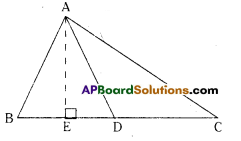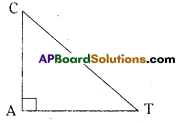AP State Syllabus AP Board 7th Class Maths Solutions Chapter 5 Triangle and Its Properties Ex 2 Textbook Questions and Answers.

## AP State Syllabus 7th Class Maths Solutions 5th Lesson Triangle and Its Properties Exercise 2Question 1.
In ΔABC, D is the midpoint of $$\overline{\mathrm{BC}}$$
(i) $$\overline{\mathrm{AD}}$$ is the ___________________
(ii) $$\overline{\mathrm{AE}}$$ is the ____________________Solution:
(i) $$\overline{\mathrm{AD}}$$ is the median
(ii) $$\overline{\mathrm{AE}}$$ is the Altitude

Question 2.
Name the triangle in which the two altitudes of the triangle are two of its sides.
Solution:
In Right angled triangle, the sides containing the right
angle are two altitudes.
In ΔCAT, ∠A = 90° and CA; AT are altitudes.Question 3.
Does a median always lie in the interior of the triangle?
Solution:
Yes, a median always lie in the interior of the triangle.Question 4.
Does an altitude always lie in the interior of a triangle’?
Solution:
No, an altitude need not always lie In the interior of a triangle.

Question 5.
(i) Write the side opposite to vertex Y in ΔXYZ.
(ii) Write the angle opposite to side $$\overline{\mathrm{PQ}}$$ in ΔPQR.
(iii) Write the vertex opposite to side $$\overline{\mathrm{AC}}$$ in ΔABC.
Solution:
i) Side opposite to vertex Y = $$\overline{\mathrm{XZ}}$$
ii) Angle opposite to side $$\overline{\mathrm{PQ}}$$ = ∠R
lii) Vertex opposite to side $$\overline{\mathrm{AC}}$$ = B